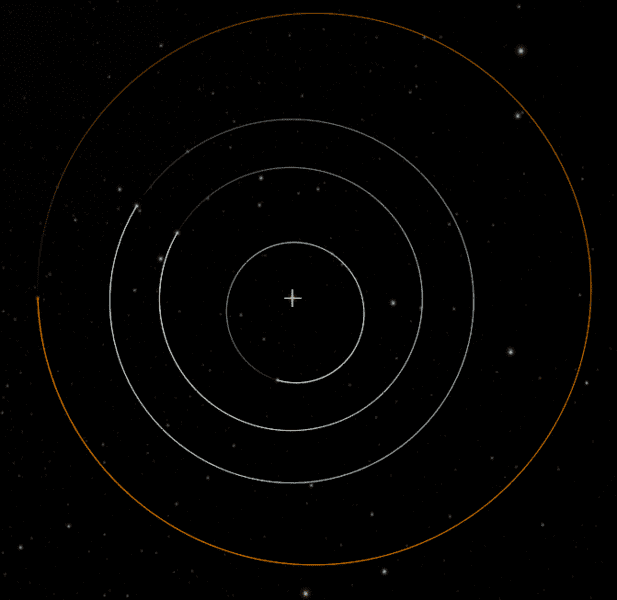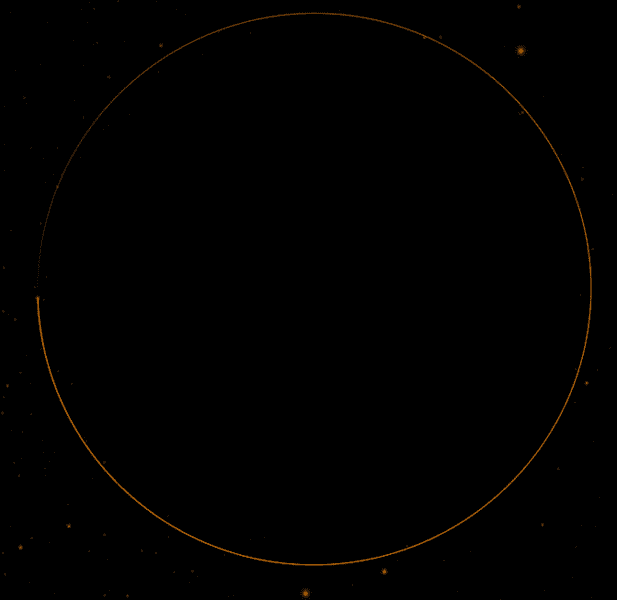# Earth's orbit around the sun -- looking for an equation

• B
• Romanko

#### Romanko

Hi, I'm looking for some help please. I'm struggling to find an equation of the Earth orbiting around the sun. I don't need to include the mass of the sun and the gravity laws, etc. Just the equation to calculate a position in x and y. Can anybody help me please?
My idea is to put the orbit inside of a software where I have coordinates x y and z, the sun being on (0,0) and the Earth orbiting around it.

Roman.

Hi, I'm looking for some help please. I'm struggling to find an equation of the Earth orbiting around the sun. I don't need to include the mass of the sun and the gravity laws, etc. Just the equation to calculate a position in x and y. Can anybody help me please?
My idea is to put the orbit inside of a software where I have coordinates x y and z, the sun being on (0,0) and the Earth orbiting around it.

Roman.
You could use the equation of an ellipse: ##\frac{x^2}{a^2}+\frac{y^2}{b^2}=1## since both semi-axis ##a,b## are known. Next you only have to shift the coordinates in a way, that the sun isn't at a focus but at ##(0,0)## instead, although it's probably easier to keep the sun at a focus point.

•Romanko
If you just want to graph the shape of the orbit, you can use
$$r = \frac{a (1-e^2)}{1+e \cos q}$$

r is the radial distance from the Sun
a is the semi-major axis of the orbit (average orbital radius)
e is the eccentricity of the orbit
q is the angle between the object and perihelion (closest approach to the Sun)

This will give results in polar coordinates which you can then convert to xy coordinates if you need to.
For the earth: a = ~ 1.496e6 km e = ~0.017 ( if you need more accurate values, you can get them from the Wikipedia article for the Earth.

If you are looking to calculate the Earth's position over time, there is no single equation method of determining this. There are methods that use iteration, where you take the output of an equation and put it back into the equation as a variable, and repeat until you get an accuracy in your answer you can live with. But these methods require the use of orbital mechanic that do make use of the mass of the Sun and the Laws of Gravity.

•Romanko
Thanks a lot Fresh and Janus, at the beginning when I put the formula into the software, I thought there was a mistake because I was seeing what looked like a circle. It was after I zoomed, and focused better when I actually could see the ellipse. This make me think that all the diagrams that I studied my whole life in school are actually an exaggeration of the ellipse!

Thanks a lot Fresh and Janus, at the beginning when I put the formula into the software, I thought there was a mistake because I was seeing what looked like a circle. It was after I zoomed, and focused better when I actually could see the ellipse. This make me think that all the diagrams that I studied my whole life in school are actually an exaggeration of the ellipse!

it's just that, while the Earth's orbit is an ellipse, it is an ellipse with very low eccentricity, so it is almost a circle.

•Romanko and fresh_42
Thanks a lot Fresh and Janus, at the beginning when I put the formula into the software, I thought there was a mistake because I was seeing what looked like a circle. It was after I zoomed, and focused better when I actually could see the ellipse. This make me think that all the diagrams that I studied my whole life in school are actually an exaggeration of the ellipse!
Here's the inner solar system out to Mars as seen from above, The Sun is marked by the cross.
Venus and Earth's orbits are pretty much impossible to distinguish from circles with the Sun at their centers.Even the more elliptical orbits of Mercury and Mars would be difficult to tell were ellipses if it weren't for their offset relative to the Sun. for example, here's Mars orbit without the sun and other planet orbits for reference:#### Attachments

•Romanko and fresh_42
Amazing, the whole solar system orbit concept that I had in my head was wrong... Thanks!